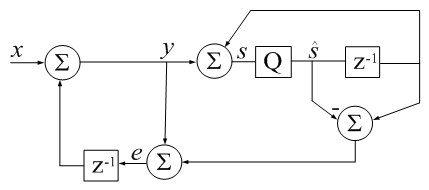# Accumulator Error Feedback

(Difference between revisions)
 Revision as of 22:50, 28 November 2017 (edit)← Previous diff Revision as of 21:43, 29 November 2017 (edit) (undo)Next diff → Line 1: Line 1: [[Image:Gleich.jpg|thumb|right|429px|CSUM() in Digital Signal Processing terms: [[Image:Gleich.jpg|thumb|right|429px|CSUM() in Digital Signal Processing terms: - z-1 is a unit delay, Q a 64-bit floating-point quantizer, + z-1 is a unit delay,
Q is a 64-bit floating-point quantizer. - qi represents error due to quantization (additive by definition). + Algebra represents neither a sequence of instructions or algorithm. Algebra represents neither a sequence of instructions or algorithm. - It is only meant to remind that an imperfect accumulator introduces noise into a series.]] + It is only meant to remind that an imperfect accumulator introduces noise into a series. +
qi represents error due to quantization (additive by definition). + ]]

function s_hat = csum(x)                                                                                                                                     function s_hat = csum(x)

## Revision as of 21:43, 29 November 2017CSUM() in Digital Signal Processing terms: z-1 is a unit delay,
Q is a 64-bit floating-point quantizer. Algebra represents neither a sequence of instructions or algorithm. It is only meant to remind that an imperfect accumulator introduces noise into a series.
qi represents error due to quantization (additive by definition).
```function s_hat = csum(x)
% CSUM Sum of elements using a compensated summation algorithm.
%
% For large vectors, the native sum command in Matlab does
% not appear to use a compensated summation algorithm which
% can cause significant roundoff errors.
%
% This Matlab code implements a variant of Kahan's compensated
% summation algorithm which often takes about twice as long,
% but produces more accurate sums when the number of
% elements is large. -David Gleich
%
% Also see SUM.
%
% % Matlab csum() Example:
% clear all
% csumv=0;  rsumv=0;
% while csumv <= rsumv
%    v = randn(13e6,1);
%    rsumv = abs(sum(v) - sum(v(end:-1:1)));
%    disp(['rsumv = ' num2str(rsumv,'%18.16f')]);
%    [~, idx] = sort(abs(v),'descend');
%    x = v(idx);
%    csumv = abs(csum(x) - csum(x(end:-1:1)));
%    disp(['csumv = ' num2str(csumv,'%18.16e')]);
% end

s_hat=0; e=0;
for i=1:numel(x)
s_hat_old = s_hat;
y = x(i) + e;
s_hat = s_hat_old + y;
e = (s_hat_old - s_hat) + y;  %calculate difference first (Higham)
end
return
```

### sorting

In practice, input sorting

```[~, idx] = sort(abs(x),'descend');
x = x(idx);
```

should begin the csum() subroutine inside to achieve the most accurate summation. That is not presented here because the commented Example (inspired by Higham) would then display false positive results. Even in complete absence of sorting, csum() can be more accurate than conventional summation by orders of magnitude.